Simplify each only using positive exponents: 2x^-3 • 4x^2 2x^4 • 4x^-3 2x^3y^-3 • 2x

Question

Simplify each only using positive exponents:
2x^-3 • 4x^2
2x^4 • 4x^-3
2x^3y^-3 • 2x

in progress 0
5 months 2021-08-27T12:56:20+00:00 1 Answers 4 views 0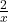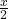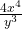Step-by-step explanation:

a. 2x^-3 • 4x^2

To solve this using only positive exponents, follow these steps:

i. Rewrite the expression in a clearer form

2x⁻³ . 4x²

ii. The position of the term with negative exponent is changed from denominator to numerator or numerator to denominator depending on its initial position. If it is at the numerator, it is moved to the denominator. If otherwise it is at the denominator, it is moved to the numerator. When this is done, the negative exponent is changed to positive.

In our case, the first term has a negative exponent and it is at the numerator. We therefore move it to the denominator and change the negative exponent to  positive as follows;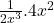iii. We then solve the result as follows;=Therefore, 2x⁻³ . 4x² =b. 2x^4 • 4x^-3

i. Rewrite as follows;

2x⁴ . 4x⁻³

ii. The second term has a negative exponent, therefore swap its position and change the negative exponent to a positive one.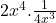iii. Now solve by cancelling out common terms in the numerator and denominator. So we have;Therefore, 2x⁴ . 4x⁻³ =c. 2x^3y^-3 • 2x

i. Rewrite as follows;

2x³y⁻³ . 2x

ii. Change position of terms with negative exponents;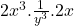iii. Now solve;Therefore, 2x³y⁻³ . 2x =